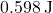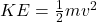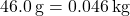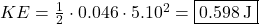## A 46.0 g golf ball is travelling with a velocity of 5.10 m/s. What is the kinetic energy of the golf ball

Question

A 46.0 g golf ball is travelling with a velocity of 5.10 m/s. What is the kinetic energy of the golf ball

in progress 0
2 months 2021-07-30T09:03:14+00:00 2 Answers 2 views 0Explanation:

The kinetic energy of a golf ball is given by.

Since the golf ball is given in grams, we must convert to kilograms, as the SI unit of mass is kilograms.Substituting given values, we have: